### Home > CALC > Chapter 4 > Lesson 4.1.2 > Problem4-19

4-19.
1. For each f(x), find its general antiderivative, F(x). Homework Help ✎

1. f(x) = −2

2.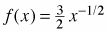3. f(x) = −3x2 + 6x

4. f(x) = 2(x + 3)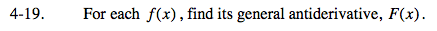Just as f '(x) is the slope function of f(x), f(x) is the slope function of F(x).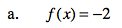If the slope function, f(x), is horizontal,then the original function, F(x), is _______________.

If f(x) is horizontal, then F(x) will be linear: F(x) = -2x + C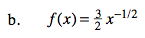Check your work by finding the derivative of F(x). Are your + and − signs correct?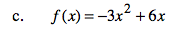For all of these, don't forget the +C.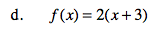If the slope function, f(x), is linear,then the original function, F(x), will be _____________________________.